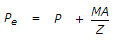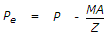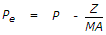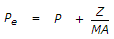# Civil Engineering - Steel Structure Design

### Exercise :: Steel Structure Design - Section 1

16.

The equivalent axial tensile load Pe, which produces an average axial tensile stress in the section equivalent to the combined stress due to axial tension P and bending M, at the extreme fibre of the section, is given by (where Z is the section modulus of the section).

 A.B.C.D.Explanation:

No answer description available for this question. Let us discuss.

17.

 A. varies in magnitude B. varies in position C. is expressed as uniformly distributed load D. All the above.

Explanation:

No answer description available for this question. Let us discuss.

18.

The most economical section for a column, is

 A. rectangular B. solid round C. flat strip D. tubular section E. hexagonal.

Explanation:

No answer description available for this question. Let us discuss.

19.

If the depth of two column sections are equal, then the column splice is provided

 A. with filler plates B. with bearing plates C. with filler and hearing plates D. none of these.

Explanation:

No answer description available for this question. Let us discuss.

20.

The distance between e.g. of compression and e.g. of tension flanges of a plate girder, is known as

 A. overall depth B. clear depth C. effective depth D. none of these.